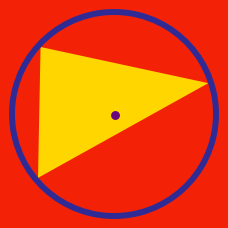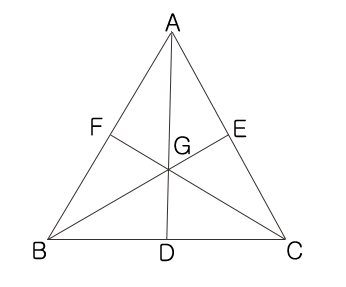Geometry

# Triangles - Centroid

The centroid is the intersection of which 3 lines in a triangle?

If we want to balance a triangle-shaped board over a pen, at which point of the triangle should the tip of the pen be placed?

Which of the following triangles has medians that do NOT intersect at one point?

Which of following triangles has medians whose intersection lies outside of the triangle?$AD, BE,$ and $CF$ are the medians of triangle $ABC,$ and $G$ is the intersection of the medians. If $AB=10, BC=12,$ and $CA=14,$ which triangle has the largest area?

×